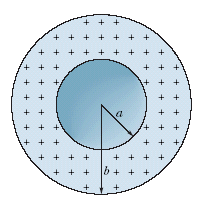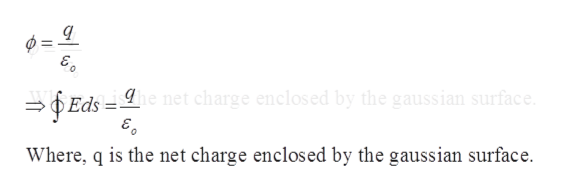# + ++ + ++ + + 1++++++++++ +++++++h ++ ++Z

Question
27 views

The figure shows a spherical shell with uniform volume charge density ρ = 2.09 nC/m3, inner radius a = 10.0 cm, and outer radius b = 2.1a. What is the magnitude of the electric field at radial distances (a) r = 0; (b) r = a/2.00, (c) r = a, (d) r = 1.50a, (e) r = b, and (f) r = 3.00b?help_outlineImage Transcriptionclose+ + + + + + + + 1 +++ +++ + ++ + + + + + + ++ h + + ++ Z fullscreen
check_circle

Step 1

Since we only answer up to 3 sub-parts, we’ll answer the first 3. Please resubmit the question and specify the other subparts (up to 3) you’d like answered.

Step 2

Gauss’s law state that the flux of the net electric field through a closed surface equals the net charge enclosed by the surface divide by electric constant.help_outlineImage Transcriptionclose= Eds=e net charge enclosed by the gaussian surface. Where, q is the net charge enclosed by the gaussian surface. fullscreen
Step 3

Since the shell is hollow inside, it has no charge if the radius is less than a.  Volume charge density is 2.09 nC/m3, and the varies as the radius increase. T...

### Want to see the full answer?

See Solution

#### Want to see this answer and more?

Solutions are written by subject experts who are available 24/7. Questions are typically answered within 1 hour.*

See Solution
*Response times may vary by subject and question.
Tagged in

### Electric Charges and Fields